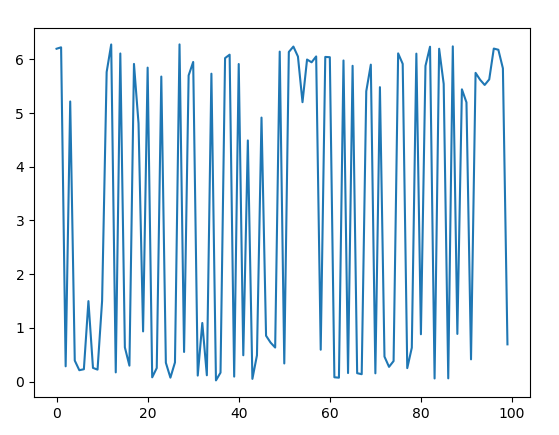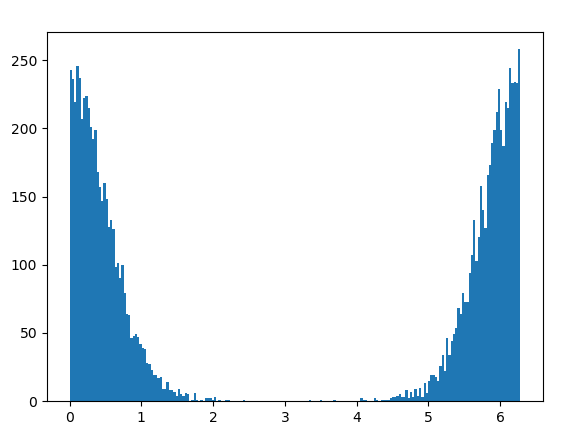# random.vonmisesvariate() function in Python

`random` module is used to generate random numbers in Python. Not actually random, rather this is used to generate pseudo-random numbers. That implies that these randomly generated numbers can be determined.

## random.vonmisesvariate()

`vonmisesvariate() ` is an inbuilt method of the `random` module. It is used to return a random floating point number with von Mises distribution or circular normal distribution.

Syntax : random.vonmisesvariate(mu, kappa)

Parameters :
mu : mean angle, expressed in radians between 0 and 2*pi
kappa : concentration parameter, greater than or equal to zero

Returns : a random von Mises distribution floating number

Example 1:

 `# import the random module ` `import` `random ` ` `  `# determining the values of the parameters ` `mu ``=` `0` `kappa ``=` `4` ` `  `# using the vonmisesvariate() method ` `print``(random.vonmisesvariate(mu, kappa)) `

Output :

`0.9429600175580171`

Example 2: We can generate the number multiple times and plot a graph to observe the von Mises distribution.

 `# import the required libraries  ` `import` `random  ` `import` `matplotlib.pyplot as plt  ` `   `  `# store the random numbers in a   ` `# list  ` `nums ``=` `[]  ` `mu ``=` `0` `kappa ``=` `4` `   `  `for` `i ``in` `range``(``100``):  ` `    ``temp ``=` `random.vonmisesvariate(mu, kappa) ` `    ``nums.append(temp)  ` `       `  `# plotting a graph  ` `plt.plot(nums)  ` `plt.show() `

Output :Example 3: We can create a histogram to observe the density of the von Mises distribution.

 `# import the required libraries  ` `import` `random  ` `import` `matplotlib.pyplot as plt  ` `   `  `# store the random numbers in a list  ` `nums ``=` `[]  ` `mu ``=` `0` `kappa ``=` `4` `   `  `for` `i ``in` `range``(``10000``):  ` `    ``temp ``=` `random.vonmisesvariate(mu, kappa)  ` `    ``nums.append(temp)  ` `       `  `# plotting a graph  ` `plt.hist(nums, bins ``=` `200``)  ` `plt.show() `

Output :Whether you're preparing for your first job interview or aiming to upskill in this ever-evolving tech landscape, GeeksforGeeks Courses are your key to success. We provide top-quality content at affordable prices, all geared towards accelerating your growth in a time-bound manner. Join the millions we've already empowered, and we're here to do the same for you. Don't miss out - check it out now!

Previous
Next